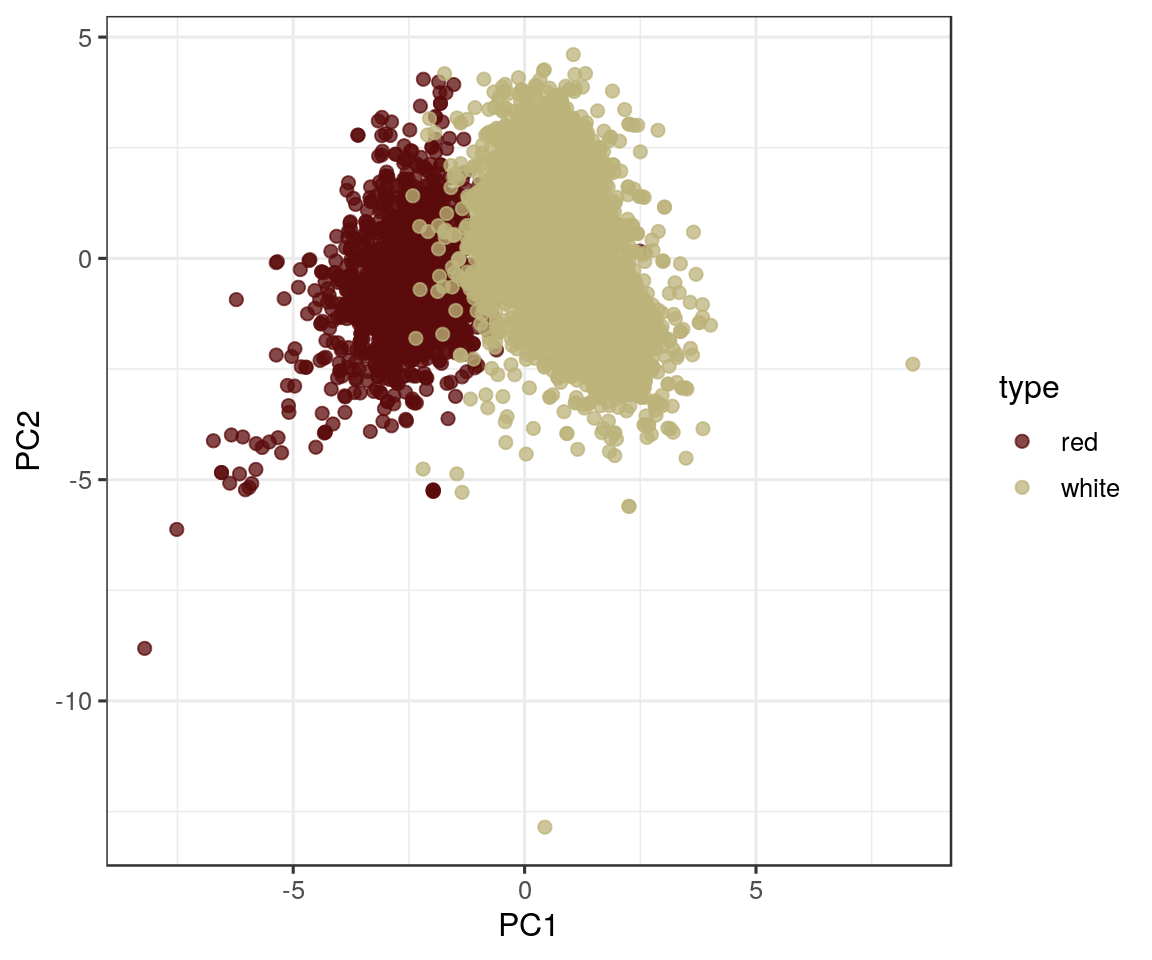## Project 2

Enter your name and EID here

This is the dataset you will be working with:

``````wine_features <-
mutate(type = as.factor(type))``````
``````## Parsed with column specification:
## cols(
##   type = col_character(),
##   quality = col_double(),
##   alcohol = col_double(),
##   pH = col_double(),
##   fixed_acidity = col_double(),
##   volatile_acidity = col_double(),
##   citric_acid = col_double(),
##   residual_sugar = col_double(),
##   chlorides = col_double(),
##   free_sulfur_dioxide = col_double(),
##   total_sulfur_dioxide = col_double(),
##   density = col_double(),
##   sulphates = col_double()
## )``````

### Part 1

Question: Can red and white wines be distinguished based on their physicochemical composition?

To answer this question, perform a principal component analysis. Make a scatterplot of PC2 vs. PC1, and a rotation matrix visualizing the influence of the input variables. Hint: You must remove all categorical variables before creating the PCA object.

Introduction: The dataset `wine_features` contains 6497 rows describing various chemical attributes of red and white wine (indicated by the `type` column) and each wine’s relative quality (indicated by the `quality`/`quality_grade` column) on a scale of 3 to 9 as graded by experts performing a blind taste test. Chemical attributes recorded for each wine include `fixed_acidity`, `volatile_acidity`, `citric_acid`, `residual_sugar`, `chlorides`, `free_sulfur_dioxide`, `total_sulfur_dioxide`, `density`, `pH`, `sulphates`, `alcohol`. Categorical descriptions of alcohol content and acidity are given by `alcohol_grade` and `acidity_grade`.

Approach: First, I will remove all non-numerical columns from the dataset (`type`, `quality_grade`, `alcohol_grade`, and `acidity_grade`). Then, I will perform a PCA on the remaining numerical columns using the functions `scale()` and `prcomp()`, and save the results in a new dataframe called `pca_data`. To evaluate whether the physicochemical properties describe the type of wine, I’ll make a scatterplot (using `geom_point()`) comparing the first and second principal components, coloring each point by type of wine. Then, I’ll make a new dataframe containing the rotation matrix and visualize the rotation matrix using `geom_segment()` and `geom_text_repel()`.

Analysis:

``````# perform PCA on `wine_features` dataset
pca <- wine_features %>%
scale() %>%
prcomp()

# get transformation data from PCA object for further analysis
pca_data <- data.frame(pca\$x, wine_features)``````
``````# color PC2 vs. PC1 scatterplot by type of wine
ggplot(pca_data, aes(x = PC1, y = PC2, color = type)) +
geom_point(alpha = 0.75, size = 2) +
scale_color_manual(values = c(wine_palette, wine_palette))````````````# capture the rotation matrix in a data frame
rotation_data <- data.frame(pca\$rotation,
variable = row.names(pca\$rotation))

# define a pleasing arrow style
arrow_style <- arrow(length = unit(0.075, "inches"),
type = "closed")

# now plot, using geom_segment() for arrows and geom_text for labels
ggplot(rotation_data) +
geom_segment(aes(xend = PC1, yend = PC2),
x = 0, y = 0,
arrow = arrow_style) +
geom_text_repel(aes(x = PC1, y = PC2, label = variable),
size = 3,
color = wine_palette,
#vjust = 0,
segment.size = 0,
set.seed(13)) +
xlim(-1., 1.) +
ylim(-1., 1.) +
coord_fixed() # fix aspect ratio to 1:1``````